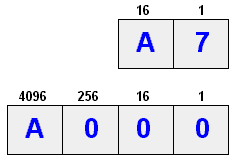# hexadecimal

Also found in: Dictionary, Thesaurus, Medical, Financial, Acronyms, Wikipedia.

## hexadecimal notation

, hexadecimal
a number system having a base 16; the symbols for the numbers 0--9 are the same as those used in the decimal system, and the numbers 10--15 are usually represented by the letters A--F. The system is used as a convenient way of representing the internal binary code of a computer
Collins Discovery Encyclopedia, 1st edition © HarperCollins Publishers 2005

## hexadecimal

[‚hek·sə′des·məl]
(mathematics)
Pertaining to a number system using the base 16. Also known as sexadecimal.
McGraw-Hill Dictionary of Scientific & Technical Terms, 6E, Copyright © 2003 by The McGraw-Hill Companies, Inc.

## hexadecimal

(mathematics)
(Or "hex") Base 16. A number representation using the digits 0-9, with their usual meaning, plus the letters A-F (or a-f) to represent hexadecimal digits with values of (decimal) 10 to 15. The right-most digit counts ones, the next counts multiples of 16, then 16^2 = 256, etc.

For example, hexadecimal BEAD is decimal 48813:

digit weight value B = 11 16^3 = 4096 11*4096 = 45056 E = 14 16^2 = 256 14* 256 = 3584 A = 10 16^1 = 16 10* 16 = 160 D = 13 16^0 = 1 13* 1 = 13 ----- BEAD = 48813

There are many conventions for distinguishing hexadecimal numbers from decimal or other bases in programs. In C for example, the prefix "0x" is used, e.g. 0x694A11.

Hexadecimal is more succinct than binary for representing bit-masks, machines addresses, and other low-level constants but it is still reasonably easy to split a hex number into different bit positions, e.g. the top 16 bits of a 32-bit word are the first four hex digits.

The term was coined in the early 1960s to replace earlier "sexadecimal", which was too racy and amusing for stuffy IBM, and later adopted by the rest of the industry.

Actually, neither term is etymologically pure. If we take "binary" to be paradigmatic, the most etymologically correct term for base ten, for example, is "denary", which comes from "deni" (ten at a time, ten each), a Latin "distributive" number; the corresponding term for base sixteen would be something like "sendenary". "Decimal" is from an ordinal number; the corresponding prefix for six would imply something like "sextidecimal". The "sexa-" prefix is Latin but incorrect in this context, and "hexa-" is Greek. The word octal is similarly incorrect; a correct form would be "octaval" (to go with decimal), or "octonary" (to go with binary). If anyone ever implements a base three computer, computer scientists will be faced with the unprecedented dilemma of a choice between two *correct* forms; both "ternary" and "trinary" have a claim to this throne.
This article is provided by FOLDOC - Free Online Dictionary of Computing (foldoc.org)

## hex

(HEXadecimal) Hexadecimal means 16, and the base 16 numbering system is used as a shorthand for representing binary numbers. Each half byte (four bits) is assigned a hex digit or letter as in the following chart with its decimal and binary equivalents. Hex values are identified with an "h" or dollar sign, thus \$A7, A7h and A7H all stand for hex A7. See hex chart and hex editor.
```    Base16   10   2Hex  Dec  Binary
0    0    0000
1    1    0001
2    2    0010
3    3    0011
4    4    0100
5    5    0101
6    6    0110
7    7    0111
8    8    1000
9    9    1001
A   10    1010
B   11    1011
C   12    1100
D   13    1101
E   14    1110
F   15    1111
```

Interpreting the HexAs decimal digits increment by 10, hexadecimal digits increment by 16. Hexadecimal is a shorthand for human readability of binary, which is the intrinsic numbering system in the computer. See binary values.

``` A7
= decimal 167 (10x16+7x1)
= binary 10100111 (128+32+4+2+1)

A000
= decimal 40,960 (10x4096)
= binary 1010000000000000 (32768+8192)
```
Copyright © 1981-2019 by The Computer Language Company Inc. All Rights reserved. THIS DEFINITION IS FOR PERSONAL USE ONLY. All other reproduction is strictly prohibited without permission from the publisher.
References in periodicals archive ?
The mean of the data bytes in decimal format was converted to hexadecimal to get an estimated average of the luminance of each image and its corresponding gradient after the pixel sorting process.
Different bits of the entered hexadecimal numbers are taken to form a binary vector (see the last process above).
Test Hexadecimal Test Hexadecimal Test data number data number data 0000 0 0100 4 1000 0001 1 0101 5 1001 0010 2 0110 6 1010 0011 3 0111 7 1011 Test Hexadecimal Test Hexadecimal data number data number 0000 8 1100 C 0001 9 1101 D 0010 A 1110 E 0011 B 1111 F TABLE 2: CEBM.
This field is no longer used, and is now always set to a hexadecimal value of FF.
The far left four-digit hexadecimal group, in the above example, includes two colons between it and the rest of the numbering scheme.
(209.) The term hexadecimal literally means "of, relating to,
In the ADS-B system, each aircraft is assigned a unique hexadecimal ICAO code, as well as your N-number, which will show up to ATC and other UAT-equipped aircraft in the system.
A particularly likely source of error that he does not mention may have arisen in the translation of numbers from hexadecimal to decimal notation, as pointed out by Philip Metzger (personal communication).
Note that, in addition to accepting a decimal value, this code value can be entered using other radixes (14) as well, such as hexadecimal (15), just remember to include the appropriate radix flag character with the value (e.g.

Site: Follow: Share:
Open / Close